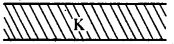# 2nd PUC Physics Series-6

#### PUC Science 2nd Year Physics Series-6 quiz

2
Created on Bywefru

2nd PUC Physics Series 6

TEST YOUR KNOWLEDGE OF SCIENCE

1 / 10

51. Two capacitors of 3 µF and 6 µF are connected in series with a battery of P.d. 12 V. The P.d. across 3µF and 6µF
capacitors respectively will be :

2 / 10

Q.52 An electric charge of 425 f µC is removed from a fully charged capacitor of capacitance 8.5µF. Its potential will be lowered by:

3 / 10

53. A dielectric is placed in between the two parallel plates of a capacitor as shown in the figure. The dielectric constant of the dielectric being K. If the initial capacity is C, then the new capacity will be:4 / 10

54. Minimum number of capacitor of 2µF each required to obtain a capacitance of 5µF will be:

5 / 10

55. When a dielectric slab is introduced between the plates of a parallel plate capacitor which remains connected to a battery then charge on the plates relative to earlier charge is :

6 / 10

56. Choose the vector physical quantity:

7 / 10

57. The surface density of charge is measure:

8 / 10

58. A polythene piece rubbed with wool is found to have a negative charge of 6.4 × 10-9c. How many electrons have been transferred during the process?

9 / 10

59. What is the number of electrons in 100 g water?

10 / 10

60. A uniform electric field of 10 NC-1 exists in the vertically downward direction. What is the increase in the electric potential asonegoes up through a height of 300 cm ?